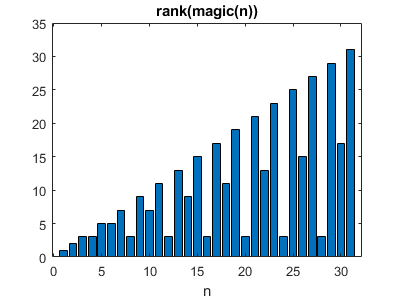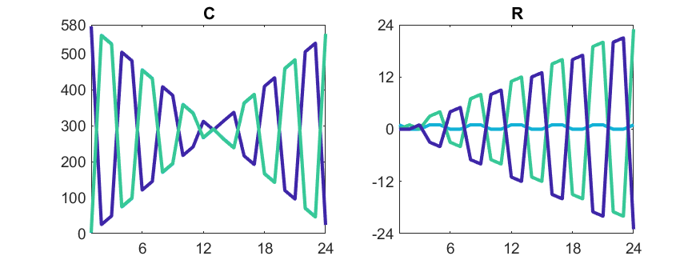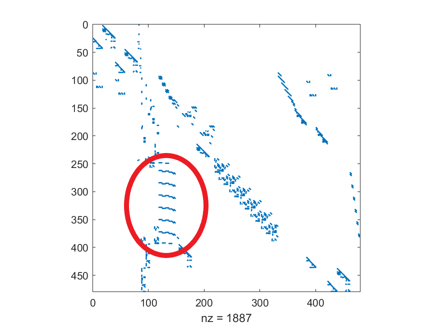# Notes on CR and west0479

My post a few days ago, Gil Strang and the CR Matrix Factorization, generated a lot of email. Here is the resulting follow-up to that post.

### Contents

#### CR via backslash

Suppose A is rank deficient and that its leading columns, C, reveals its rank. Then the factorization

C*R = A

is a systen of linear, overdetermined equations for the elements of R. How do you solve such systems? "Divide" both sides by C.

R = C\A

Backslash will find the least squares solution to this overdetermined system. If A actually does have such a factorization, the least squares solution will be an exact solution and the resulting R will be in what we could call the " canonical reduced row echelon form" of A. It will have the zero rows deleted and the identity matrix in the leading rows and columns.

When the elements of A are integers, you can remove the roundoff fuzz and recover them with

round(C*R)

If you don't know the rank, you can use backslash in a loop.

    type cr_backslash

function [C,R] = cr_backslash(A)
for r = 1:size(A,2)
C = A(:,1:r);
R = C\A;
if round(C*R) == A
break
end
end
end



Here's an example

   A = [ 0  3 12  6
1  2  9  7
2  1  6  8
3  0  3  9 ];

[C,R] = cr_backslash(A)

round(C*R)

C =

0     3
1     2
2     1
3     0

R =

1.0000    0.0000    1.0000    3.0000
0.0000    1.0000    4.0000    2.0000

ans =

0     3    12     6
1     2     9     7
2     1     6     8
3     0     3     9



cr_backslash works on the magic squares.

    small_fig
for n = 1:31
M = magic(n);
[C,R] = cr_backslash(M);
r(n) = size(C,2);
end
bar(r)
title('rank(magic(n))')
xlabel('n')#### CR explained

Iain Duff suggested that I offer more explanation for the plots of C and R generated by magic squares.

Here is code for magic(n) when n is a multiple of four.

  J = repmat([0 1 1 0],n/4)
K = (J' == J)
A = reshape(1:n^2,n,n)';
B = A;
A(K) = 0
B(~K) = 0;
B = rot90(B,2)
M = A + B

Here is the output when n = 8 with zeros replaced by dots. The pattern in K is responsible for the patterns we will see in plots of C and R.

J =
   .     1     1     .     .     1     1     .
K =
   1     .     .     1     1     .     .     1
.     1     1     .     .     1     1     .
.     1     1     .     .     1     1     .
1     .     .     1     1     .     .     1
1     .     .     1     1     .     .     1
.     1     1     .     .     1     1     .
.     1     1     .     .     1     1     .
1     .     .     1     1     .     .     1
A =
.     2     3     .     .     6     7     .
9     .     .    12    13     .     .    16
17     .     .    20    21     .     .    24
.    26    27     .     .    30    31     .
.    34    35     .     .    38    39     .
41     .     .    44    45     .     .    48
49     .     .    52    53     .     .    56
.    58    59     .     .    62    63     .
B =
  64     .     .    61    60     .     .    57
.    55    54     .     .    51    50     .
.    47    46     .     .    43    42     .
40     .     .    37    36     .     .    33
32     .     .    29    28     .     .    25
.    23    22     .     .    19    18     .
.    15    14     .     .    11    10     .
8     .     .     5     4     .     .     1
M =
  64     2     3    61    60     6     7    57
9    55    54    12    13    51    50    16
17    47    46    20    21    43    42    24
40    26    27    37    36    30    31    33
32    34    35    29    28    38    39    25
41    23    22    44    45    19    18    48
49    15    14    52    53    11    10    56
8    58    59     5     4    62    63     1

C is the first three columns of M and R is the canonical reduced row echelon form of M.

C =
  64     2     3
9    55    54
17    47    46
40    26    27
32    34    35
41    23    22
49    15    14
8    58    59

R =

   1     0     0     1     1     0     0     1
0     1     0     3     4    -3    -4     7
0     0     1    -3    -4     4     5    -7

Columns 2 and 3 of C differ by +1 or -1, so in plot(C) they are on top of each other in the dark blue. Column 1, in blue green, complements the other two columns.

Row 1 of R is just 0 or 1; it is the light blue in plot(R ), The other two rows of R complement each other.

Here are the plots when n = 24.#### west0479 explained

Art Westerberg retired from Carnegie Mellon in 2003, but that does't stop him from explaining the chemical engineering going on in west0479.

West0479 is from a model of a distillation column. Such a device is a stack of repeated units called trays. The fingerprints of these trays are all over a spy plot of west0479. The modeling equations for a tray only reference variables within the tray. To create a column, one "connects" the outflow and inflow of fluid and vapor from each tray to the tray above it and the one below it. This creates the equations that have just two nonzero coefficients, a +1 or -1 and a smaller value.

rref is not a sparse solver; it just plows across the matrix. When its drop tolerance, tol, is larger than one, the connections are ignored, resulting in an echelon form that is sparser than the original, and a lousy approximation. When tol is smaller than one, the vital connections are included, but the fill-in effectively connects each tray to all the other trays. This also results in a poor approximation.#### west0479 iterated

John Gilbert tells us that today systems of equations involving west0479 are readily solved by direct methods, but the eleven orders of magnitude variation in the coefficients causes iterative methods to struggle.

#### west0479 inspires

Tim Davis is inspired by west0479. A "favicon" is a small icon for a website. See the one in the upper left hand corner of this old web page: Tim's Matrices. Be sure to see Tim's Gallery, the Horror Matrix at Tim's Poetry, and Cleve's Corner.

Published with MATLAB® R2020a

|

### コメント

コメントを残すには、ここ をクリックして MathWorks アカウントにサインインするか新しい MathWorks アカウントを作成します。